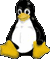# Shell Script Tutorial

### Logic - IF/THEN/ELSE

Let's look at the if/then/else construct in a Bash shell script and see how to control the flow of a script with conditional logic. The general form of if/then/else is shown here, with the actual syntax shown in boldface and the parts you must supply in normal type:
```
if [ condition is true ]; then
execute these commands
else
execute those commands
fi```
The else clause is optional, but you must end the construct with the fi command. You can also have nested if clauses by using the elif command like this:
```
if [ condition1 is true ]; then
execute these commands
elif [ condition2 is true ]; then
execute these commands
else
execute those commands
fi```
So what kind of conditions can we test for? If you're dealing with numbers, here are the conditional expressions you can use. In other words, any of these expressions can go inside the brackets on the if or elif statement:

num1 -eq num2 True if num1 equals num2.

num1 -ne num2 True if num1 is not equal to num2.

num1 -lt num2 True if num1 is less than num2.

num1 -gt num2 True if num1 is greater than num2.

num1 -le num2 True if num1 is less than or equal to num2.

num1 -ge num2 True if num1 is greater than or equal to num2.

str1 = str2 True if str1 and str2 are identical.

str1 != str2 True if str1 and str2 are not identical.

-n str1 True if str1 is not null (length is greater than zero).

-z str1 True if str1 is null (length is zero).

-f somefile True if somefile exists and is an ordinary file.

-d somefile True if somefile exists and is a directory.

-s somefile True if somefile contains data (the size is not zero).

-r somefile True if somefile is readable.

-w somefile True if somefile is writable.

-x somefile True if somefile is executable.

And finally, here are the logical operators, for performing tests that involve and, or, and not conditions.

cond1 -a cond2 True if both cond1 and cond2 are true.

cond1 -o cond2 True if either cond1 or cond2 is true.

! cond1 True if cond1 is false.

Example for if/then/else

Use this syntax to run executable command in IF condition
if [ "`command`" ]; then

```
if [ \$carprice -gt 20000 ]; then
echo 'Too rich for my blood.'
else
echo 'Can you get that model in blue?'
fi

if [ \$maker = 'Buick' ]; then
echo 'Have you driven a Ford lately?'
fi

if [ -r \$1 -a -s \$2 ]; then
echo "The \$1 file is readable and contains data."
fi

if [ "`/bin/ping -c1 hk.yahoo.com | grep ttl`" ]; then
echo "Ping yahoo OK"
else
echo "Ping yahoo is failed"
fi

if [ -n "`ps axf | grep httpd`" ]; then
echo "httpd is running..."
else
echo "httpd is stopped."
fi

## To check if a directory exists:

if [ -d "\$DIRECTORY" ]; then
# Control will enter here if \$DIRECTORY exists
fi

## Or to check if a directory doesn't exist:

if [ ! -d "\$DIRECTORY" ]; then
# Control will enter here if \$DIRECTORY doesn't exist
fi

```Server is hosted by Alanstudio
Linux Operating System

Recommend screen resolution 1024 x 768 / IE / FireFox